How Cheenta works to ensure student success?
Explore the Back-Story

# Test of Mathematics Solution Subjective 55 - Partition of a set of functionsThis is a Test of Mathematics Solution Subjective 55 (from ISI Entrance). The book, Test of Mathematics at 10+2 Level is Published by East West Press. This problem book is indispensable for the preparation of I.S.I. B.Stat and B.Math Entrance.

Also visit: I.S.I. & C.M.I. Entrance Course of Cheenta

## Problem

For a finite set A, let |A| denote the number of elements in set A.

(a) Let F be the set of all functions f:{1, 2, ... , n} --> {1, 2, ... , k}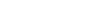satisfying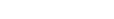for every i,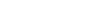. Show that |F| =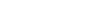.

## Solution

The function f may take the element 1 in domain to one of the k numbers in codomain (k choices for 1). Since f(2) cannot equal f(1), we have (k-1) choices for 2 to go. Similarly f(3) cannot equal f(2) (but it can equal f(1)) so there are k-1 choices for 3 and so on. In total the number of functions possible equals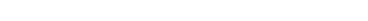.

(b) Let c(n,k) denote the number of functions in F such that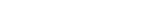. Then show that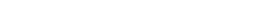Solution: We consider the following partition of the set of functions F:
(1) functions in which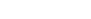(2) functions in which f(n) = f(1)
Note the above partition is well defined as they are mutually exclusive and exhaustive. Functions of type (1) are given by c(n, k) and functions of type two is given by c(n-1, k) because if f(n) = f(1), f(n-1) cannot equal f(1) as F contains functions for whichAs functions of type 1 and type 2
makes up all the functions in F we have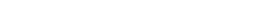.

(c) Using part (b) or otherwise prove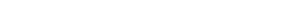Solution: We use induction on part (b). Suppose. Then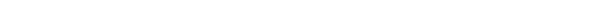This is a Test of Mathematics Solution Subjective 55 (from ISI Entrance). The book, Test of Mathematics at 10+2 Level is Published by East West Press. This problem book is indispensable for the preparation of I.S.I. B.Stat and B.Math Entrance.

Also visit: I.S.I. & C.M.I. Entrance Course of Cheenta

## Problem

For a finite set A, let |A| denote the number of elements in set A.

(a) Let F be the set of all functions f:{1, 2, ... , n} --> {1, 2, ... , k}satisfyingfor every i,. Show that |F| =.

## Solution

The function f may take the element 1 in domain to one of the k numbers in codomain (k choices for 1). Since f(2) cannot equal f(1), we have (k-1) choices for 2 to go. Similarly f(3) cannot equal f(2) (but it can equal f(1)) so there are k-1 choices for 3 and so on. In total the number of functions possible equals.

(b) Let c(n,k) denote the number of functions in F such that. Then show thatSolution: We consider the following partition of the set of functions F:
(1) functions in which(2) functions in which f(n) = f(1)
Note the above partition is well defined as they are mutually exclusive and exhaustive. Functions of type (1) are given by c(n, k) and functions of type two is given by c(n-1, k) because if f(n) = f(1), f(n-1) cannot equal f(1) as F contains functions for whichAs functions of type 1 and type 2
makes up all the functions in F we have.

(c) Using part (b) or otherwise proveSolution: We use induction on part (b). Suppose. Then### Knowledge Partner